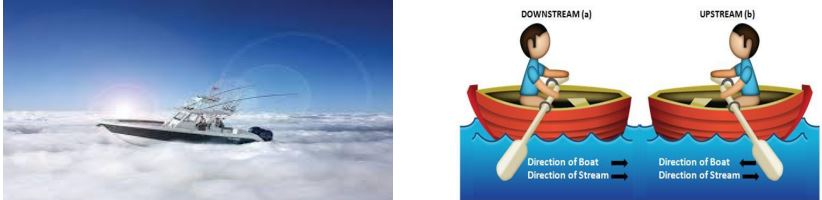# CBSE Board Exam 2021: Check Case Study Based Question Bank for Class 10 Maths Chapter 4 (Released By CBSE)

CBSE Class 10 Maths Case Study Questions for Chapter 4 - Quadratic Equations are released by the board. Practice all these questions to perform in your upcoming CBSE Class 10 Maths Exam 2021.

Created On: Apr 8, 2021 19:00 ISTCBSE Class 10 Maths Case Study Questions for Chapter 4

CBSE Class 10 Maths paper which is scheduled for 2nd June, 2021, will include questions based on case study. This is for the first time that the Maths paper will have case study questions. For students to not have any problem in solving these new type of questions, the board has provided a question bank based on case study. All these questions are quite useful to prepare for the upcoming board exam.

We have provided below the questions for CBSE Class 10 Maths Chapter 4 - Quadratic Equations. All the questions are provided with answers. Students must practice all the case study based questions to prepare well for their Maths exam.

Case Study Questions for Class 10 Maths Chapter 4 - Quadratic Equations

CASE STUDY 1:

Raj and Ajay are very close friends. Both the families decide to go to Ranikhet by their own cars. Raj’s car travels at a speed of x km/h while Ajay’s car travels 5 km/h faster than Raj’s car. Raj took 4 hours more than Ajay to complete the journey of 400 km.1. What will be the distance covered by Ajay’s car in two hours?

a) 2(x + 5)km

b) (x – 5)km

c) 2(x + 10)km

d) (2x + 5)km

Answer: a) 2(x + 5)km

2. Which of the following quadratic equation describe the speed of Raj’s car?

a) x2 – 5x – 500 = 0

b) x2 + 4x – 400 = 0

c) x2 + 5x – 500 = 0

d) x2 – 4x + 400 = 0

Answer: c) x2 + 5x – 500 = 0

3. What is the speed of Raj’s car?

a) 20 km/hour

b) 15 km/hour

c) 25 km/hour

d) 10 km/hour

Answer: a) 20 km/hour

4. How much time took Ajay to travel 400 km?

a) 20 hour

b) 40 hour

c) 25 hour

d) 16 hour

Answer: d) 16 hour

CASE STUDY 2:

The speed of a motor boat is 20 km/hr. For covering the distance of 15 km the boat took 1 hour more for upstream than downstream.1. Let speed of the stream be x km/hr. then speed of the motorboat in upstream will be

a) 20 km/hr

b) (20 + x) km/hr

c) (20 – x) km/hr

d) 2 km/hr

Answer: c) (20 – x)km/hr

2. What is the relation between speed ,distance and time?

a) speed = (distance )/time

b) distance = (speed )/time

c) time = speed x distance

d) speed = distance x time

Answer: b) distance = (speed )/time

3. Which is the correct quadratic equation for the speed of the current?

a) x2 + 30x − 200 = 0

b) x2 + 20x − 400 = 0

c) x2 + 30x − 400 = 0

d) x2 − 20x − 400 = 0

Answer: c) x2 + 30x − 400 = 0

4. What is the speed of current ?

a) 20 km/hour

b) 10 km/hour

c) 15 km/hour

d) 25 km/hour

Answer: b) 10 km/hour

5. How much time boat took in downstream?

a) 90 minute

b) 15 minute

c) 30 minute

d) 45 minute

Answer: d) 45 minute

Comment ()

6 + 7 =
Post